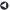### Statistical Services OfferedBack to List of Services

#### Regression Analysis and Forecasting

Regression Analysis is a technique for determining the significance of the predictor (or independent) variables (the X's) in explaining the variability or behavior of the response (or dependent) variable (the Y's) and for predicting its values given the values of the predictor variables. For linear regression analysis, the assumptions are: the response variable (the Y's) is continuous; the mean of the Y's is accurately modeled by a linear function of the X's; the random error term has a normal distribution with a mean of zero and a constant variance; and the errors are independent. One method for verifying these assumptions is to plot the residuals versus the predicted values and versus the predictor variables.

Polynomial regression is a type of multiple linear regression where powers of variables (e.g., quadratic and/or cubic terms) and cross-product (or interaction) terms are included in the model. Polynomial regression models are linear in the parameters (or coefficients) and are therefore a type of linear regression. It is generally recommended to build hierarchically well-formulated models: a model which includes a variable to a power should also include all lower powers of the variable and a model which has a cross product term should also include each of the individual variable terms.

Multicollinearity is a common problem with polynomial models. As higher order terms are added to the model, they tend to be highly correlated with one another. A technique for minimizing this problem is to recenter the variables by subtracting the sample mean from each observed value.

Nonlinear Regression: A linear regression model is linear in the parameters (or coefficients). This means there is only one parameter in each term of the model and each parameter is a multiplicative constant of the independent variables(s) of that term. A nonlinear model is nonlinear in the parameters and cannot be linearized by a transformation of the equation. Nonlinear models cannot be solved explicitly, and iterative methods must be used to estimate the parameters. Specification of the starting values for the parameters is a critical part of this process. In SAS software, the NLin procedure is used for nonlinear regression models.

Poisson Regression: As mentioned previously, the general linear model assumes: the response variable (the Y's) is continuous; the mean of the Y's is accurately modeled by a linear function of the X's; and the random error term has a normal distribution. Generalized linear models extend the general linear model in two ways: 1.) the distribution of the random error can come from the family of exponential distributions (e.g., binomial and Poisson) rather than only the normal distribution as assumed in the general linear model; and 2.) the relationship between the mean of the Y's and linear function of the X's (the link function) can be any monotonic function rather than only the identity function as prescribed by the general linear model.

A Poisson regression model with no random effects belongs to the class of generalized linear regression models and is fit in SAS software with the GenMod procedure. If the model includes random effects, it belongs to the class of generalized linear regression mixed models and is fit with the GLIMMIX procedure which became available as of SAS 9.1.

Poisson regression is an application of the generalized linear model in which the response variable is a count, the distribution is Poisson, and the link function is the natural logarithm. The Poisson regression model is used extensively in many different fields. Phenomena which involve a count of events in which large counts would be rare are candidates for Poisson regression analysis. The distribution of these counts tends to be skewed to the right, usually with a large number of zero occurrences. Examples are number of: bank failures, loan defaults, doctor's visits, injuries in a work place, and recreational trips.